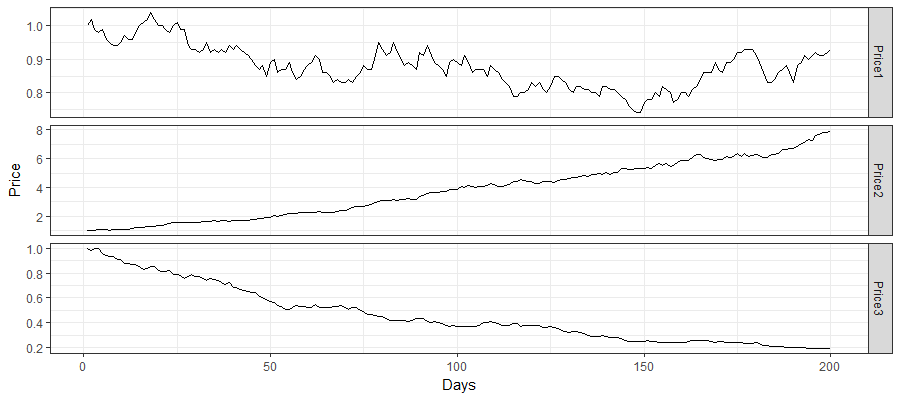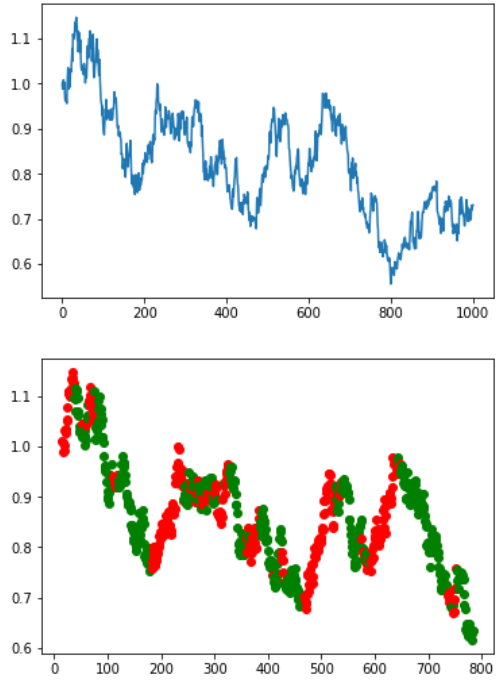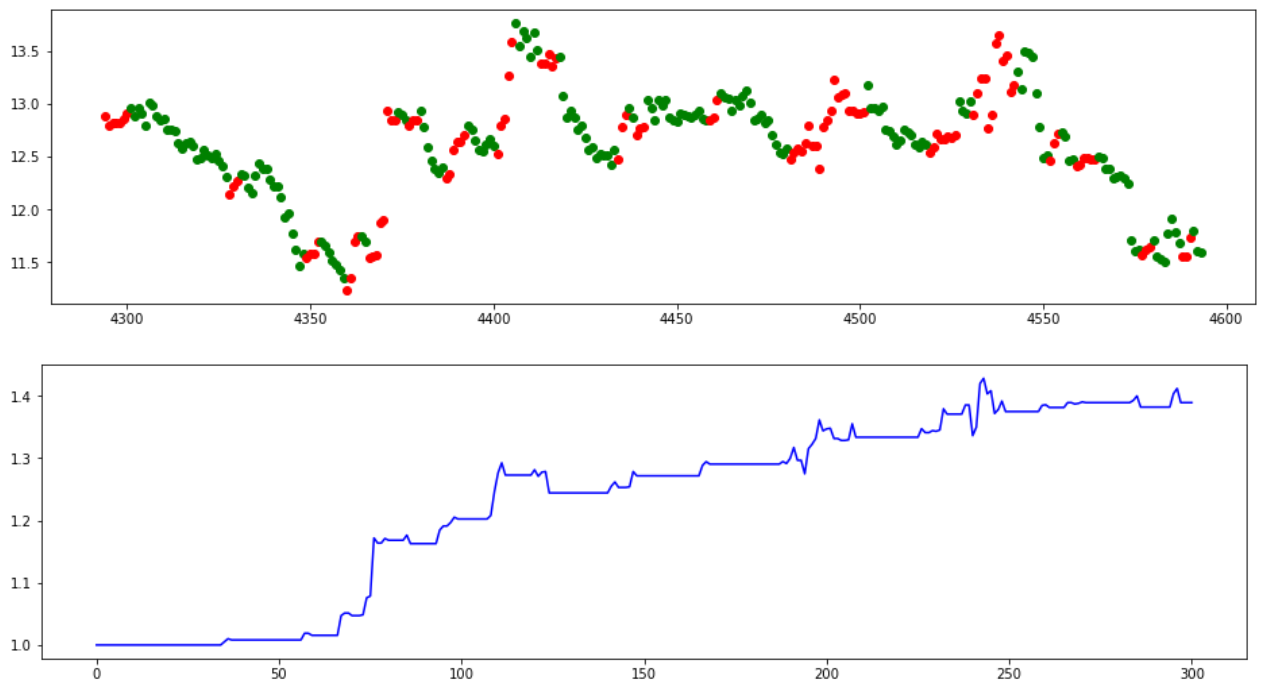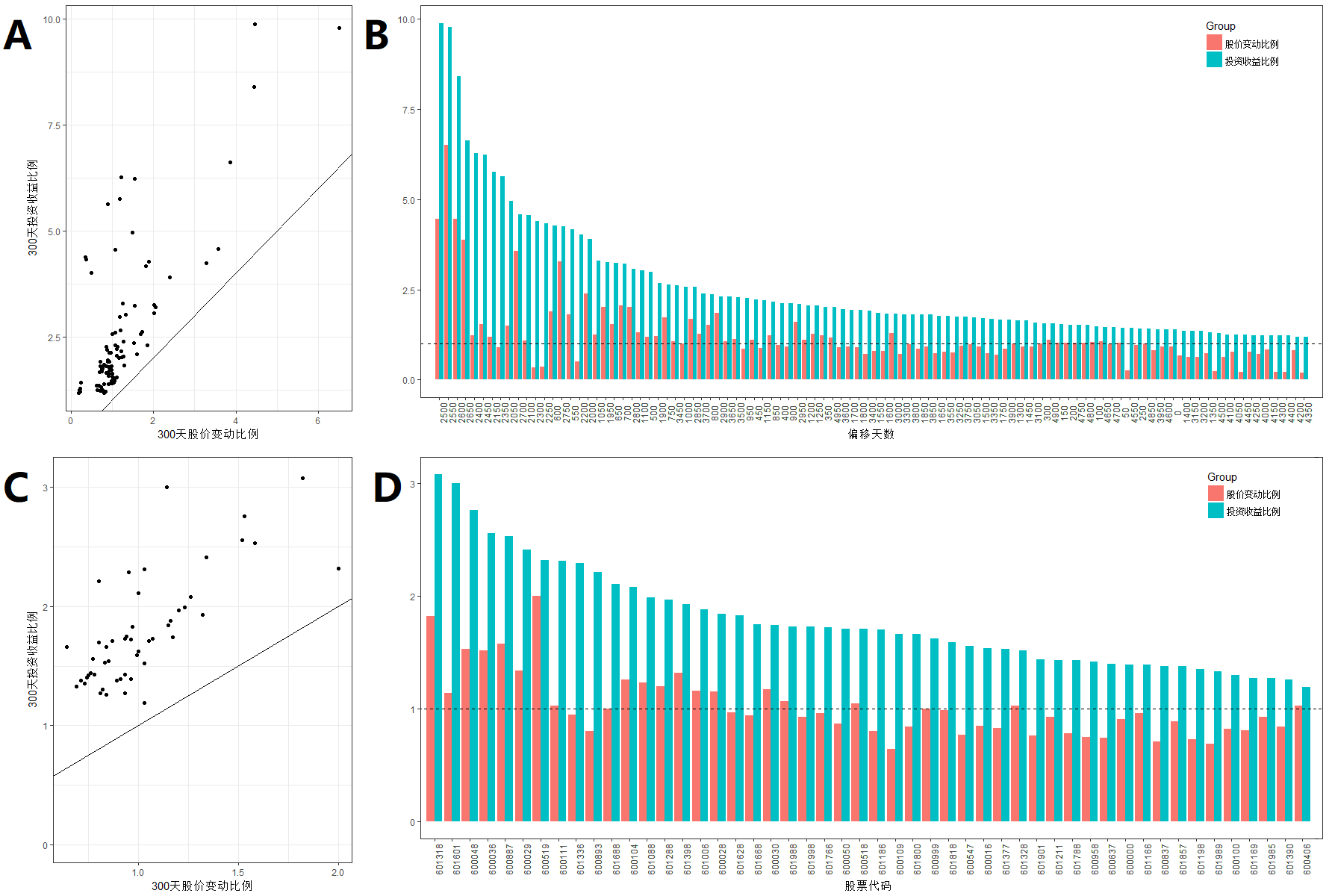第一章 量化交易与隐马尔科夫模型

量化交易

量化交易的特点

1. 纪律性。根据模型的运行结果进行决策，而不是凭感觉。纪律性既可以克制人性中贪婪、恐惧和侥幸心理等弱点，也可以克服认知偏差，且可跟踪。
2. 系统性。具体表现为“三多”。一是多层次，包括在大类资产配置、行业选择、精选具体资产三个层次上都有模型；二是多角度，定量投资的核心思想包括宏观周期、市场结构、估值、成长、盈利质量、分析师盈利预测、市场情绪等多个角度；三是多数据，即对海量数据的处理。
3. 套利思想。定量投资通过全面、系统性的扫描捕捉错误定价、错误估值带来的机会，从而发现估值洼地，并通过买入低估资产、卖出高估资产而获利。
4. 概率取胜。一是定量投资不断从历史数据中挖掘有望重复的规律并加以利用；二是依靠组合资产取胜，而不是单个资产取胜。

量化交易的应用——算法交易

1. 被动型算法交易，也称结构型算法交易。该交易算法除利用历史数据估计交易模型的关键参数外，不会根据市场的状况主动选择交易时机和交易的数量，而是按照一个既定的交易方针进行交易。该策略的的核心是减少滑价（目标价与实际成交均价的差）。被动型算法交易最成熟，使用也最为广泛，如在国际市场上使用最多的成交加权平均价格（VWAP）、时间加权平均价格（TWAP）等都属于被动型算法交易。
2. 主动型算法交易，也称机会型算法交易。这类交易算法根据市场的状况作出实时的决策，判断是否交易、交易的数量、交易的价格等。主动型交易算法除了努力减少滑价以外，把关注的重点逐渐转向了价格趋势预测上。
3. 综合型算法交易，该交易是前两者的结合。这类算法常见的方式是先把交易指令拆开，分布到若干个时间段内，每个时间段内具体如何交易由主动型交易算法进行判断。两者结合可达到单纯一种算法无法达到的效果。

隐马尔科夫模型

解码问题： Viterbi算法

• 初始(i=0):
• 递归(i=1···L):
• 终止:
• 回溯(i=1···L):

def Viterbi(O,LM,CM):
LM = np.log(LM)
CM = np.log(CM)
pi=[[1,1]]
for i in range (1,len(O)):
pi.append([0,0])
if O[i] == "R":
pi[i] = LM+max(pi[i-1]+CM,pi[i-1]+CM)
pi[i] = LM+max(pi[i-1]+CM,pi[i-1]+CM)
else:
pi[i] = LM+max(pi[i-1]+CM,pi[i-1]+CM)
pi[i] = LM+max(pi[i-1]+CM,pi[i-1]+CM)

if pi[len(O)-1] >= pi[len(O)-1]:
H = "U";pr_H = "U"
else:
H = "D";pr_H = "D"

for i in range (len(O)-2,-1,-1):
if pr_H == "D":
if pi[i]+CM > pi[i]+CM:
pr_H="U"
else:
pr_H="D"
if pr_H=="U":
if pi[i]+CM > pi[i]+CM:
pr_H="U"
else:
pr_H="D"
H=pr_H+H

return H

• 初始(i=0):
• 递归(i=1···L):
• 终止:

学习问题： Baum-Welch算法

(1)隐式序列已知时的估计

(2)隐式序列未知时的估计：Baum-Welch和Viterbi训练

• 起始：
• 任取模型参数$a_{kl}$和$e_k(b)$
• 递归:
• 将所有的A和E变量设置于他们的伪计数$r$（或0）
• 对每条序列$j=1···n$:
用前向算法对序列$j$计算$f_k(i)$
用后向算法对序列$j$计算$b_k(i)$
将序列j的贡献添加到A和E中
• 计算新的参数模型
• 计算模型的新对数似然
• 终止：
• 如果对数似然变化小于某个设定的阈值
• 如果迭代次数超过最大值，则终止

第二章 实验结果与讨论

 若收盘价-开盘价 +0.13 +0.21 -0.43 +0.54 -0.22 -0.23 -0.11 +0.02 -0.05 记录的显式序列 R R G R G G G R G

模拟股价测试

基于几何布朗运动的人工股价随机函数

import matplotlib.pyplot as plt
import numpy as np

def Price(f=0.02,u=0,t=200):
v = f*np.random.randn(t)+u
P=
for i in range (t-1):
P.append(P[i]*np.exp(v[i]))
plt.plot(P)
plt.show()
return P,v

\#价格水平通道曲线
Price()

\#价格上升通道曲线
Price(u=0.01)

\#价格下降通道曲线
Price(u=-0.01)隐马尔可夫模型简单测试上证50真实数据回测

 浦发银行 (600000) 民生银行 (600016) 中国石化 (600028) 南方航空 (600029) 中信证券 (600030) 招商银行 (600036) 保利地产 (600048) 中国联通 (600050) 同方股份 (600100) 上汽集团 (600104) 国金证券 (600109) 北方稀土 (600111) 国电南瑞 (600406) 康美药业 (600518) 贵州茅台 (600519) 山东黄金 (600547) 东方明珠 (600637) 海通证券 (600837) 伊利股份 (600887) 中航动力 (600893) 东方证券 (600958) 招商证券 (600999) 大秦铁路 (601006) 中国神华 (601088) 兴业银行 (601166) 北京银行 (601169) 中国铁建 (601186) 东兴证券 (601198) 国泰君安 (601211) 农业银行 (601288) 中国平安 (601318) 交通银行 (601328) 新华保险 (601336) 兴业证券 (601377) 中国中铁 (601390) 工商银行 (601398) 中国太保 (601601) 中国人寿 (601628) 中国建筑 (601668) 华泰证券 (601688) 中国中车 (601766) 光大证券 (601788) 中国交建 (601800) 光大银行 (601818) 中国石油 (601857) 方正证券 (601901) 中国核电 (601985) 中国银行 (601988) 中国重工 (601989) 中信银行 (601998)单一股票定时测试

import csv
Data = []
with open('600000.csv','rb') as ff:
for row in reader:
Data.append(row)
O = ""
Price = []
Rate0 = []
for i in range(len(Data)-2):
if(Data[i+2]!="null" and Data[i+1]!="null"):
Price.append(Data[i+1])
Rate0.append(float(Data[i+2])/float(Data[i+1]))
if (float(Data[i+2])-float(Data[i+1])>=0):
O = O + "R"
else:
O = O + "G"

import matplotlib.pyplot as plt
days = 300
Hdline = Viterbi(O,[[0.6, 0.4], [0.4, 0.6]],[[0.6, 0.4], [0.4, 0.6]])
fig, ax = plt.subplots(figsize=(16, 4))
for i in range (len(O)-days-1,len(O)-1):
if Hdline[i] == "U":
ax.plot([i],[Price[i]],'ro')
elif Hdline[i] == "D":
ax.plot([i],[Price[i]],'go')
plt.show()
\#图像化显示计算出的涨跌区间

fig, ax = plt.subplots(figsize=(16, 4))
Price_my = 
flag = False
for i in range (len(O)-days,len(O)):
if flag == False:
Price_my.append(Price_my[len(Price_my)-1]*1.0)
else:
Price_my.append(Price_my[len(Price_my)-1]*Rate0[i])
if Hdline[i-1] == "U" and  Hdline[i] == "D":
flag = False
elif Hdline[i-1] == "D" and Hdline[i] == "U":
flag = True
ax.plot(Price_my,'b')
plt.show()
\#依照此涨跌去购买的收益曲线

单一股票时间变动测试

import csv

def F(x):
if x == 'U':
return 0
else:
return 1

def f(x):
if x == 'R':
return 0
else:
return 1
def right(O,Hdline,days,x):
t_num=0
f_num=0
for i in range(len(O)-days-x*50,len(O)-x*50):
if f(O[i]) == F(Hdline[i]):
t_num=t_num+1
else:
f_num=f_num+1
return float(t_num)/float(f_num+t_num)

def main(filename,x):
#print("%-15s %-15s %-15s %-15s"%("偏移天数","投资收益","实际涨幅","准确率"))
Data = []
with open(filename,'rb') as ff:
for row in reader:
Data.append(row)
O = ""
Price = []
Rate0 = []
for i in range(len(Data)-2):
if(Data[i+2]!="null" and Data[i+1]!="null"):
Price.append(Data[i+1])
Rate0.append(float(Data[i+2])/float(Data[i+1]))
if (float(Data[i+2])-float(Data[i+1])>=0):
O = O + "R"
else:
O = O + "G"
Hdline = Viterbi(O,[[0.6, 0.4], [0.4, 0.6]],[[0.6, 0.4], [0.4, 0.6]])

Price_my = 1
flag = False
days = 300
for i in range (len(Price)-days-x*50,len(Price)-x*50):
if flag == False:
Price_my = Price_my*1.0
else:
Price_my = Price_my*Rate0[i]
if Hdline[i-1] == "U" and  Hdline[i] == "D":
flag = False
elif Hdline[i-1] == "D" and Hdline[i] == "U":
flag = True
print("%-11.6s %-11.2f %-11.2f %-11.2f"%(x*50,round(Price_my,2),
float(Price[len(Price)-1-x*50])/float(Price[len(Price)-1-days-x*50]),
right(O,Hdline,days,x)))

print("%-15s %-15s %-15s %-15s"%("偏移天数","投资收益","实际涨幅","准确率"))
for i in range(100):
main('600000.csv',i)
 偏移天数 投资收益 实际涨幅 准确率 0 1.39 0.91 0.77 50 1.43 1.03 0.77 100 1.48 1.05 0.78 150 1.54 1.03 0.81 200 1.53 1.02 0.81 ··· ··· ··· ··· 4750 1.52 1.02 0.80 4800 1.51 1.03 0.81 4850 1.42 1.01 0.79 4900 1.56 1.11 0.80 4950 2.01 1.17 0.81

上证50样本300天测试

def right(O,Hdline):
t_num=0
f_num=0
for i in range(len(O)-days,len(O)):
if f(O[i]) == F(Hdline[i]):
t_num=t_num+1
else:
f_num=f_num+1
return float(t_num)/float(f_num+t_num)

def result(f_list):
print("%-15s %-15s %-15s %-15s"%("股票代码","投资收益","实际涨幅","准确率"))
for i in range (len(f_list)):
main(f_list[i])

def main(filename):
Data = []
with open(filename,'rb') as ff:
for row in reader:
Data.append(row)
O = ""
Price = []
Rate0 = []
for i in range(len(Data)-2):
if(Data[i+2]!="null" and Data[i+1]!="null"):
Price.append(Data[i+1])
Rate0.append(float(Data[i+2])/float(Data[i+1]))
if (float(Data[i+2])-float(Data[i+1])>=0):
O = O + "R"
else:
O = O + "G"

days = 300
Hdline = Viterbi(O,[[0.6, 0.4], [0.4, 0.6]],[[0.6, 0.4], [0.4, 0.6]])

Price_my = 1
flag = False
for i in range (len(Price)-days,len(Price)):
if flag == False:
Price_my = Price_my*1.0
else:
Price_my = Price_my*Rate0[i]
if Hdline[i-1] == "U" and  Hdline[i] == "D":
flag = False
elif Hdline[i-1] == "D" and Hdline[i] == "U":
flag = True
print("%-11.6s %-11.2f %-11.2f %-11.2f"%(filename,round(Price_my,2),
float(Price[len(Price)-1])/float(Price[len(Price)-1-days]),right(O,Hdline)))
result(['600000.csv','600016.csv','600028.csv','600029.csv','600030.csv',
'600036.csv','600048.csv','600050.csv','600100.csv','600104.csv',
'600109.csv','600111.csv','600406.csv','600518.csv','600519.csv',
'600547.csv','600637.csv','600837.csv','600887.csv','600893.csv',
'600958.csv','600999.csv','601006.csv','601088.csv','601166.csv',
'601169.csv','601186.csv','601198.csv','601211.csv','601288.csv',
'601318.csv','601328.csv','601336.csv','601377.csv','601390.csv',
'601398.csv','601601.csv','601628.csv','601668.csv','601688.csv',
'601766.csv','601788.csv','601800.csv','601818.csv','601857.csv',
'601901.csv','601985.csv','601988.csv','601989.csv','601998.csv'])
 股票代码 投资收益 实际涨幅 准确率 股票代码 投资收益 实际涨幅 准确率 600000 1.39 0.91 0.77 601169 1.27 0.81 0.80 600016 1.54 0.85 0.79 601186 1.70 0.80 0.75 600028 1.84 1.15 0.80 601198 1.35 0.73 0.73 600029 2.41 1.34 0.76 601211 1.43 0.93 0.78 600030 1.74 1.17 0.76 601288 1.97 1.20 0.83 600036 2.56 1.52 0.81 601318 3.08 1.82 0.77 600048 2.76 1.53 0.81 601328 1.52 1.03 0.79 600050 1.71 0.87 0.84 601336 2.29 0.95 0.78 600100 1.30 0.82 0.85 601377 1.53 0.83 0.77 600104 2.08 1.26 0.80 601390 1.26 0.84 0.73 600109 1.66 0.64 0.79 601398 1.93 1.32 0.80 600111 2.31 1.03 0.75 601601 3.00 1.14 0.83 600406 1.19 1.03 0.82 601628 1.83 0.97 0.76 600518 1.71 1.05 0.79 601668 1.75 0.94 0.76 600519 2.32 2.00 0.79 601688 2.11 1.00 0.80 600547 1.56 0.77 0.81 601766 1.72 0.96 0.75 600637 1.40 0.74 0.77 601788 1.43 0.78 0.80 600837 1.38 0.71 0.75 601800 1.66 0.84 0.78 600887 2.53 1.58 0.81 601818 1.59 0.99 0.82 600893 2.21 0.80 0.79 601857 1.38 0.89 0.73 600958 1.42 0.75 0.75 601901 1.44 0.76 0.79 600999 1.62 1.00 0.77 601985 1.27 0.93 0.79 601006 1.88 1.16 0.81 601988 1.73 1.07 0.82 601088 1.99 1.23 0.82 601989 1.33 0.69 0.86 601166 1.39 0.96 0.79 601998 1.73 0.93 0.79

局限性分析

实际问题

• 股票交易手续费：股票交易手续费就是指投资者在委托买卖证券时应支付的各种税收和费用的总和。由印花税、佣金、过户费等组成。 1. 印花税：投资者在买卖成交后支付给财税部门的税收，需缴纳成交金额的1‰ 。2008年9月19日至今由向双边征收改为向出让方单边征收。受让者不再缴纳印花税。 2. 券商交易佣金：最高不超过成交金额的3‰，但是最低5元起。值得注意的是单笔交易佣金不满5元时，按5元收取。 3. 过户费：这是指股票成交后，更换户名所需支付的费用。从2015年8月1日起已经更改为上海和深圳都进行收取，此费用按成交金额的0.002%收取。
• 股票交易规则：股票的交易存在着一些规则，在这些规则是制约下，其实我们一般不会在我们预想的时间点用我们预想的价格买入或者卖出。 1. 瞬时性：股票的成交价格取决于买卖双方之间的平衡。事实上每一瞬时的价格是已经成交的单笔价格，而不是我的实际成交价格。为了满足在特定的时间买入，我不得不选择让利成交，这将减少我的收益。 2. 大众心态：在模型中你总能在一个价格点上买到无限多或者卖出无限多的股票，而现实中，你面对的是和你一样的众多投资者。他们也在评估是否应该买入，是否可以卖出。所以当人们看好行情时，很多人愿意让利，用高一点的价格买入，于是你不得不一步步提高自己的买入价格。反之当后市不被看好时，恐慌心理将导致价格快速的下降，甚至到无法抛售的境地。

引用

1 Baum, L. E.; Petrie, T. (1966). “Statistical Inference for Probabilistic Functions of Finite State Markov Chains”. The Annals of Mathematical Statistics. 37 (6): 1554–1563. doi:10.1214/aoms/1177699147. Retrieved 28 November 2011.

1 Baum, L. E.; Eagon, J. A. (1967). “An inequality with applications to statistical estimation for probabilistic functions of Markov processes and to a model for ecology”. Bulletin of the American Mathematical Society. 73 (3): 360. doi:10.1090/S0002-9904-1967-11751-8. Zbl 0157.11101.

1 Baum, L. E.; Sell, G. R. (1968). “Growth transformations for functions on manifolds”. Pacific Journal of Mathematics. 27 (2): 211–227. doi:10.2140/pjm.1968.27.211. Retrieved 28 November 2011.

1 Baum, L. E.; Petrie, T.; Soules, G.; Weiss, N. (1970). “A Maximization Technique Occurring in the Statistical Analysis of Probabilistic Functions of Markov Chains”. The Annals of Mathematical Statistics. 41: 164. doi:10.1214/aoms/1177697196. JSTOR 2239727. MR 0287613. Zbl 0188.49603.

1 Baum, L.E. (1972). “An Inequality and Associated Maximization Technique in Statistical Estimation of Probabilistic Functions of a Markov Process”. Inequalities. 3: 1–8.

1 Stratonovich, R.L. (1960). “Conditional Markov Processes”. Theory of Probability and its Applications. 5 (2): 156–178. doi:10.1137/1105015.

1 Thad Starner, Alex Pentland. Real-Time American Sign Language Visual Recognition From Video Using Hidden Markov Models. Master’s Thesis, MIT, Feb 1995, Program in Media Arts

1 B. Pardo and W. Birmingham. Modeling Form for On-line Following of Musical Performances. AAAI-05 Proc., July 2005.

1 Satish L, Gururaj BI (April 2003). “Use of hidden Markov models for partial discharge pattern classification”. IEEE Transactions on Dielectrics and Electrical Insulation.

 Pieczynski, Wojciech (2007). “Multisensor triplet Markov chains and theory of evidence”. International Journal of Approximate Reasoning. 45: 1–16. doi:10.1016/j.ijar.2006.05.001.

 Boudaren et al., M. Y. Boudaren, E. Monfrini, W. Pieczynski, and A. Aissani, Dempster-Shafer fusion of multisensor signals in nonstationary Markovian context, EURASIP Journal on Advances in Signal Processing, No. 134, 2012.

 Lanchantin et al., P. Lanchantin and W. Pieczynski, Unsupervised restoration of hidden non stationary Markov chain using evidential priors, IEEE Trans. on Signal Processing, Vol. 53, No. 8, pp. 3091-3098, 2005.

 Boudaren et al., M. Y. Boudaren, E. Monfrini, and W. Pieczynski, Unsupervised segmentation of random discrete data hidden with switching noise distributions, IEEE Signal Processing Letters, Vol. 19, No. 10, pp. 619-622, October 2012.

 “stock Definition”. Investopedia. Retrieved 25 February 2012

 “Understanding Stock Prices: Bid, Ask, Spread”. Youngmoney.com. Retrieved 2010-02-12.